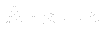Previous: Under-Relaxation Up: TCFD Solvers Next: SIMPLE Algorithm
This is an automatically generated documentation by LaTeX2HTML utility. In case of any issue, please, contact us at info@cfdsupport.com.

## System of Linear Equations

Finite Volume Method converts the system of differential equations to the system of linear equations:Such a linear algebra problem can be solved with following methods implemented in OpenFOAM:

Linear system solver method:

• GAMG (Geometric-Algebraic Multi-Grid) for both symmetric and asymmetric matrices
• PBiCG (Preconditioned Biconjugate Gradient ) for asymmetric matrices
• PCG (Preconditioned Conjugate Gradient) for symmetric matrices
• smoothSolver (solver using a smoother for both symmetric and asymmetric matrices )
• ICCG (Incomplete Cholesky preconditioned PCG solver, i.e. PBiCG with DIC )8.2
• BICCG (Diagonal Incomplete LU preconditioned PBiCG solver, i.e. PCG with DILU)8.3

Method preconditioner:

• DILU (Diagonal Incomplete LU decomposition)
• DIC (Diagonal incomplete-Cholesky) for symmetric matrices
• FDIC (Faster diagonal incomplete-Cholesky) for symmetric matrices
• diagonal (Diagonal)
• GAMG (Geometric-Algebraic Multi-Grid)
• none (No preconditioning)
• DICGaussSeidel, GaussSeidel, nonBlockingGaussSeidel, symGaussSeidel (for symmetric matrices)

For more details see e.g. .